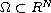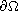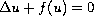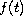Electron. J. Diff. Eqns., Vol. 2009(2009), No. 90, pp. 1-15.

### Second-order boundary estimates for solutions to singular elliptic equations Claudia Anedda

Abstract:
Letbe a bounded smooth domain. We investigate the effect of the mean curvature of the boundaryin the behaviour of the solution to the homogeneous Dirichlet boundary value problem for the singular semilinear equation. Under appropriate growth conditions onasapproaches zero, we find an asymptotic expansion up to the second order of the solution in terms of the distance fromto the boundary.

Submitted June 17, 2009. Published July 30, 2009.
Math Subject Classifications: 35B40, 35B05, 35J25.
Key Words: Elliptic problems; singular equations; second order boundary approximation.

Show me the PDF file (251 KB), TEX file, and other files for this article.Claudia Anedda Dipartimento di Matematica e Informatica Universitá di Cagliari Via Ospedale 72, 09124 Cagliari, Italy email: canedda@unica.it